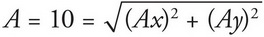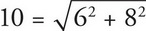# AP Physics 1 Question 185: Answer and Explanation

### Test Information

Question: 185

5. The magnitude of vector A is 10. Which of the following could be the components of A ?

• A. Ax = 5, Ay = 5
• B. Ax = 6, Ay = 8
• C. Ax = 7, Ay = 9
• D. Ax = 10, Ay = 10The only answer choice with components that satisfy this equation is (B):.